# What dividend ratios to use to evaluate dividend stocks?

Posted at Sept. 7, 2022, 6 p.m.

## How to evaluate stocks that pay dividends as an investor?

Dividend ratios are one of the key ways to evaluate and find the best dividend stocks. There are a few different types of dividend ratios, but the two most important ones are the dividend yield and the company's dividend payout ratio. But to get the best of the best we offer a lot more metrics, which we explain in this chapter. Do not be afraid, we are focused on the main indicators, explain everything as simple as possible and by the way, our tool calculates everything and ranks all the stocks on that metrics for you.

Categories of indicators we use and explain in this chapter:

Fundamental indicators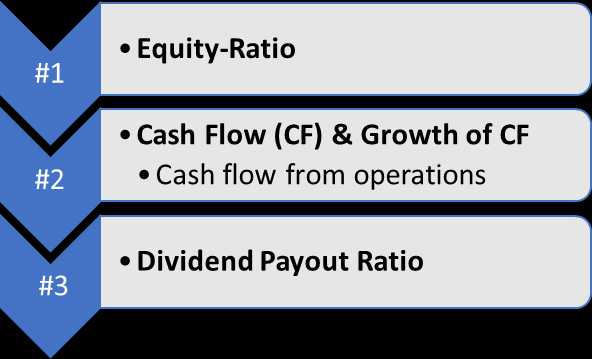Explicitly focused dividend ratios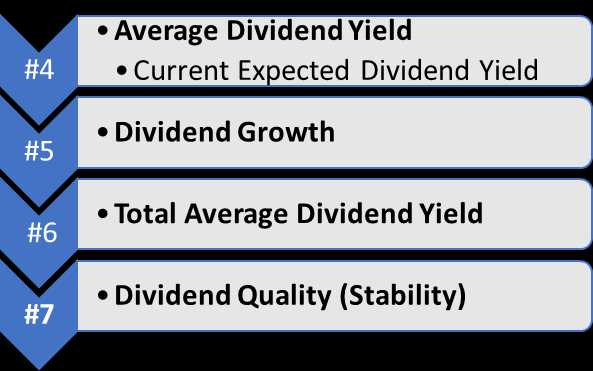Technical and risk Indicators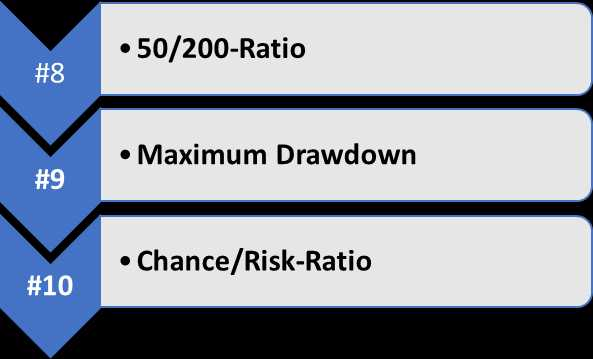## # 1 of Dividend Ratio - The Equity-Ratio

A company's equity ratio is a measure of its financial leverage, calculated by dividing its total equity by its total assets. The higher the ratio, the more equity the company has relative to its assets, and the lower the ratio, the more debt the company has relative to its equity. The equity ratio is important because it can provide insights into a company's dividend policies and how much risk it is taking on. For example, a company with a high equity ratio may be more likely to pay dividends to shareholders, while a company with a low equity ratio may be more likely to reinvest profits to finance growth. In either case, the equity ratio can give investors an idea of what to expect from a company in terms of dividend payments and risk.

Attention: The comparison between stocks of different industries is not as meaningful. An investor should compare dividend paying stocks in an industry to decide which stock is the better investment opportunity and to figure out what is the typical average in that industry.

## # 2 of Dividend Ratio - The Cash Flow (CF) and its Average Growth

First of all, we have to differentiate 4 subcategories:

• Free cash flow (FCF) is the cash a company generates after accounting for operating expenses and capital expenditures. In other words, it's the money a company has available to pay dividends.
• Cash flow from financing activities is the cash generated from a company's debt and equity financing. This includes money received from issuing new shares of stock, as well as money borrowed from lenders or debt repayment.
• Cash flow from investing activities is the cash generated from a company's investment activities. This includes money earned from interest and dividends, as well as profits from the sale of investments.
• Cash flow from operations is the cash generated from a company's day-to-day business activities. This includes money generated from sales, as well as money spent on things like employee salaries and rent.

Each of these measures is important in understanding a company's ability to generate cash. And when considering dividend stocks, it's especially important to look at the free cash flow and cash flow from operations. This will give you a good indication of whether the company has enough money available to pay its dividend obligations.

Attention: We are mainly focused on the CF from operations to make the stocks comparable to each other. Other platforms use the FCF. This approach is not as good as it sounds. Just because a company has a lot of money left in free cash flow tells nothing about how it comes from. Let's say a company's healthiness is not as good and its workload is around 50%, the CF from operations is negative. It would sell some property and show a plus in CF from investing activities. In the end, the company would maybe have a positive free cash flow. So it seems like everything is ok, but the weakness is shown in the CF from operations.
Note: The CF from operations should show an average growth - the more, the better compared to its competitors in the industry. We calculate the average by considering the last 5 years.

## # 3 of Dividend Ratio - The Dividend Payout Ratio

We already mentioned, that and why we use the cash flow from operations, which is one component to calculate the dividend payout ratio on this site. The other number that is taken into account is the paid dividends. The dividend payout ratio formula:

Dividend Payout Ratio = Paid Dividends / CF From Operations
Note: High dividend payout ratios, especially higher payout ratios compared to the average of the industry should be a warning signal - the less, the better compared to its competitors.

## # 4 of Dividend Ratio - The Dividend Yield

The dividend yield is a performance metric that dividend investors use to evaluate dividend stocks. This indicator is simply the ratio of a dividend stock's annual dividend payments to its current stock price. For example, if a dividend stock trades for \$100 per share and pays \$5 per share in dividends annually, its dividend yield would be 5%. The dividend yield is important for dividend investing because it provides a way to measure the potential return on the investment. When dividend yields are high, it means that dividend investors can potentially earn a higher return on their investment. Conversely, when dividend yields are low, it means that they may not earn as high of a return. Therefore, investors should always be on the lookout for stocks with high dividend yields.

Attention: Special dividends are one-time dividend payments that are not part of a company's regular dividend cycle. They are often paid out when a company has excess cash on hand, or when it wants to return some of its profits to shareholders. While special dividends can be a nice bonus for investors of dividend investing, they should not be the primary factor when selecting dividend stocks. Instead, investors should focus on companies with strong fundamentals and a history of consistent dividend payments. So our ratios do not consider special dividends.

### How do we calculate the average dividend yield?

To get an idea of what is the company paying normally as dividends, we take a look at the last ten years - if we have a data history that long. As we already said, we do not consider special dividend situations. After all, each year we take a look at how high a dividend per share to its average share price was. In the end, we calculate the overall average.

The goal is to calculate a high-quality indicator with no possible and surprising expectations - The dividend yield of a company under normal conditions without any fluctuation around the price speculations or special business or industry situations.

Tip: As an investor be more focused on this metric instead of the expected dividend yield.

### As informational - the expected dividend yield

But sure, we also show you the expected dividend yield. It is calculated by the announced dividend payment per share for the current year divided by the current stock price. The announced dividend payment is in the future and a special dividend may be included.

dividend current year / current stock price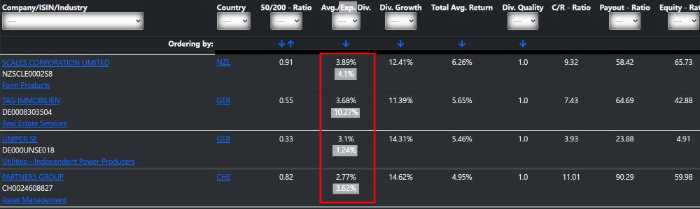Tip: If the broader market is going down, the expected dividend is rising because of the falling stock prices. So in an overall down-trending market, the expected dividend is a good indicator to filter for an attractive dividend stock. In this situation, the average dividend yield is a supporting metric that indicates sustainability. In an overall good market, it is more important to have a look at the average dividend yield.

## # 5 of Dividend Ratio - The Dividend Growth

This ratio is the percentage increase in a company's dividend payout from one year to the next. It is calculated by dividing the current year's dividend by the previous year's dividend and then expressing that result as a percentage. For example, if a company's dividend payments increased from \$1 per share to \$1.50 per share, the growth would be 50%.

Note: A company with strong fundamentals and a history of increasing its dividend payout is usually a good investment. A company that consistently fails to grow its dividend is usually not a good investment - even worse it can be a signal of a dividend cut.

### How do we calculate the ratio?

We go one step further and we calculate the standardized dividend around the average dividend of the last 10 years and divide it by the considered years of paid dividends. As a result, we can try to argue for future growth.

## # 6 of Dividend Ratio - The Total Average Dividend Yield

In general, this ratio is simply the average of a dividend yield and its dividend growth rate in a given timeframe. It is calculated by adding a company's dividend yield to its growth rate and expressing that result as a percentage.

What is needed to calculate that simply:

1. Dividend yield + its growth
2. Extrapolating it in the future, for example, 10 years
3. Calculating the average per year from the extrapolation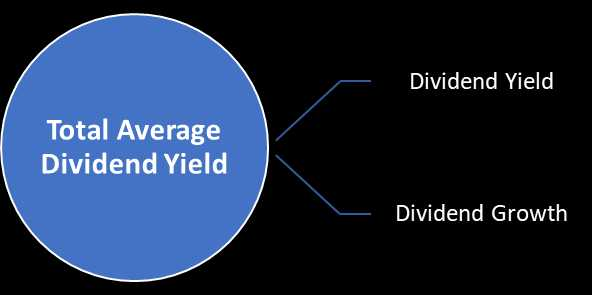Note: The total average dividend yield is a good way to measure a company's overall dividend performance and to compare dividend paying stocks without knowing the yield or the growth of the dividends themselves. But you should still keep an eye on the details because it can keep you away from pitfalls. We wrote about that in the section "High Dividend or Dividend Growth Investing: Which is Better? - The dividend sweet spot".

## # 7 of Dividend Ratio - The Dividend Quality (Stability)

With the dividend quality, we measure the stability of dividends per share in the last 10 years. If the dividend rose each year, the ratio would be at its highest result, so it is 1. If the dividends fluctuate but if average dividends of 10 years, 5 years, and 3 years are still on the top of the 0.8st quantile then we still rate the dividend quality with 1.

The quantiles we use to measure dividend quality respectively stability:

• Quantile of 0.8 = Rating is 1
• Quantile of 0.6 = Rating is 0.5
• Quantile of 0.4 = Rating is 0
• Quantile of 0.2 = Rating is -0.5
• Less = Rating is -1

### What is statistical quantile and how is it calculated?

Statistical quantiles are used to divide a given series of numbers into groups, or "quantiles". The calculation of quantiles is a statistical process that involves dividing a series of numbers into equal-sized groups. There are several different methods for calculating quantiles, but the most common is the median split. A median split divides a series of numbers into two groups: those below the median and those above the median. In data analysis, quantiles can be used to identify outliers and to understand the distribution of data.

## # 8 of Dividend Ratio - The 50/200-Ratio of Simple-Moving-Average (SMA)

The 50 SMA is calculated by taking the average closing price of a stock over the past 50 days. If the 50 SMA is rising, it indicates that the price of the stock is also rising. Conversely, if the 50 SMA is falling, it indicates that the stock's price is also falling. The 50 SMA is a popular indicator among traders because it can be used to generate buy and sell signals. For example, if the 50 SMA crosses above the 200 SMA, it is considered a bullish signal and traders may buy the stock. Similarly, if the 50 SMA crosses below the 200 SMA, it is considered a bearish signal, and traders may sell the stock.

In general, we use the simple moving averages because a long-term dividend investor is not interested in today's price fluctuation. The more days are used in calculating the simple moving average, the more stable can be argued if the price trend is going up or down.

A longer and shorter simple moving average can be compared to each other:

50/200-Ratio = 50 SMA / 200 SMA

#### Possible interpretations of the 50/200-Ratio

• 50 SMA > 200 SMA - 50/200-Ratio > 1

The short-term trend is rising. If the long-term trend is also up then it is a supporting argument for a bullish stock. If the 200 SMA is falling, the stock may be in a correction mode.

The higher the ratio above 1 the higher the probability that the price is falling back to its average respectively continuing the long-term short trend.

• 50 SMA less than 200 SMA - 50/200-Ratio < 1

The short-term trend is falling. If the long-term trend is also going down then it is a supporting argument for a bearish stock. If the 200 SMA is rising, the stock may be in a correction mode.

The lower the ratio below 1 the higher the probability that the price is rising back to its average respectively continuing the long-term long trend.

#### Overall 50/200-Ratio as a market trend indicator

The 50/200-Ratio is even more meaningful when it is calculated for the market. We have a growing list of over 1,000 dividend stocks and calculate this ratio for each stock. The average of all the 50/200-Ratios creates our market trend indicator - the current economic environment.

• Market trend indicator > 50

The overall market is bullish and as a result, investors should have a look at stocks with a 50/200-Ratio less than 1 with a rising 200 SMA. So you can find stocks which are in a correction and under the market circumstances are cheap.

• Market trend indicator < 50

The overall market is bearish and as a result, investors should have a look at stocks with a 50/200-Ratio greater than 1 and maybe also with a rising 200 SMA. So you can find stocks that are outperforming and under the market circumstances are strong.

#### The 50/200-Ratio as an industry trend indicator

A low trending industry in an overall bullish market could be a signal, that this industry is getting disrupted, so take care of this.

A ratio greater than 1 in an overall bearish market shows the relative strength of the industry and stocks in the industry in bearish markets should be considered to be invested in.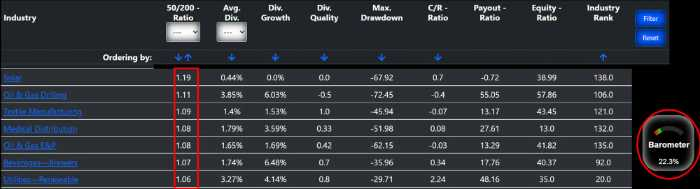#### Approach for the 50/200-Ratio

Generally, compare this ratio from stock to its industry. Additionally, the market trend shows the fundamental situation in which you would like to invest in.

1. Have a look at the market trend indicator and keep in mind how to act in the specific market situation.
2. Select your stock to invest in and compare it to the competitor's list and the industry itself.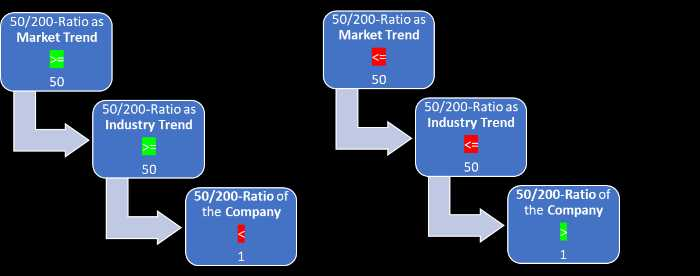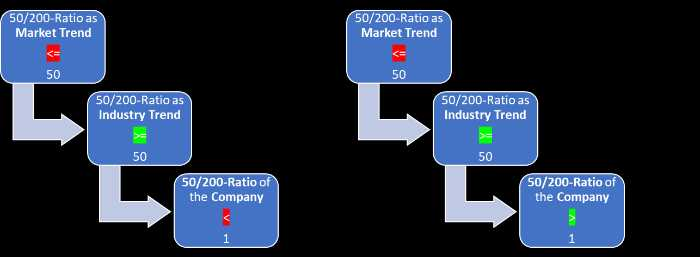## # 9 of Dividend Ratio - Maximum Drawdown

A drawdown is a peak-to-trough decline during a specific period for an investment. A drawdown is usually quoted as the percentage between the peak and the trough.

For example, consider an initial investment of \$100 that loses 20% of its value and then recovers to its original level. The peak would be \$100, the trough would be \$80, and the drawdown would be 20%.

The maximum drawdown in percent is simply the largest peak-to-trough decline during a specific period for an investment. The maximum drawdown is a measure of an investment's risk. It is used to assess how much investment can lose before it reaches its bottom. The goal for an investor is, to find the best income opportunity whit the lowest risk and the best yield for that risk.

## # 10 of Dividend Ratio - The Chance-to-Risk Ratio (C/R-Ratio)

The chance-to-risk ratio is an investment's potential return to its maximum drawdown. It is calculated by dividing the potential return by the maximum drawdown.

For example, if an investment has a potential return of 10% and a maximum drawdown of 5%, then the chance-to-risk ratio would be 2. What does that look like in the app: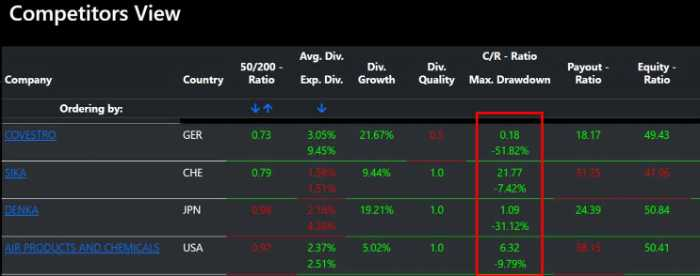The chance-to-risk ratio is a measure of an investment's risk-adjusted return. It is used to assess how much return investment can generate for each unit of risk. The goal for an investor is, to find the best income opportunity with the highest chance-to-risk ratio.

Note: As a dividend investor the C/R-Ratio is not as important as other metrics are, because:
1. We do not speculate what stock price will be in the future to measure or anticipate a book gain or loss
2. We use this metric to have a feeling that the chance to earn money (dividend and the growth of the dividend) does have a low risk on the other hand (a possible book loss). So the C/R-Ratio shows us the potential compensation of the maximum drawdown.

## Conclusion

You now have an idea and the arguments why using the metrics explained in the chapter. If it comes to dividend investing, other metrics like price to earnings ratio, earnings growth, net income, and many more will cross the path. They are nice to have but not essential - so keep less and stay focused.